﻿ Digital Circuits and Systems - Circuits i Sistemes Digitals (CSD) - EETAC - UPCBachelor's Degree in Telecommunications Systems and in Network EngineeringContent

## P1: Analysis of simple circuits based on logic gates

 Resources in lectures and labs: L1.1, L1.2, L1.3, Lab1.1, Lab1.2 Project objectives

Highlighted analysis project: Circuit_C

1. Specifications (What do I have to do?)

Analysis: deduce the truth table of circuit in Fig. 1 using the four analysis methods proposed in Fig. 3 and compare solutions. Designing equivalent circuits is discussed in the next complementary page.Fig. 1. Example Circuit_C to analyse.

Each analysis method is an independent project with all four sections.Fig. 2. Organise your analysis project following this sequence.

Fig 3 shows the general concept map including up to four methods for analysing simple combinational circuits.Fig. 3. Proposed analysis methods (Visio). The concept map combining analysis and design (Visio).

Other tutorial circuits based on logic gates:

- Other assignments (analysis problems A1.1, A1.2, ...)

2. Method 1 planning

Planning means organising and discussing how to proceed to reach solutions. A flow chart of sequential operations will be necessary to explain what to do, how to do it and when.

- Choose as circuit simulator: draw/capture the circuit schematic in Proteus and run a simulation.

- In CSD we never start a project or a simulation from scratch, but we copy and adapt from similar exercises found here in this digsys web. For example, use files to copy and adapt from .

- Select a library of a classic technology, for instance LS-TTL or CMOS series 4000.

- Try all the possible input combinations to fill in and complete the truth table.

Project location:

C:\CSD\P1\Circuit_C\Proteus\(files)

3. Method 1 development

Developing means executing a given plan to achieve a circuit solution. Fig. 4 shows an example of Proteus capture for Circuit_C.pdsprj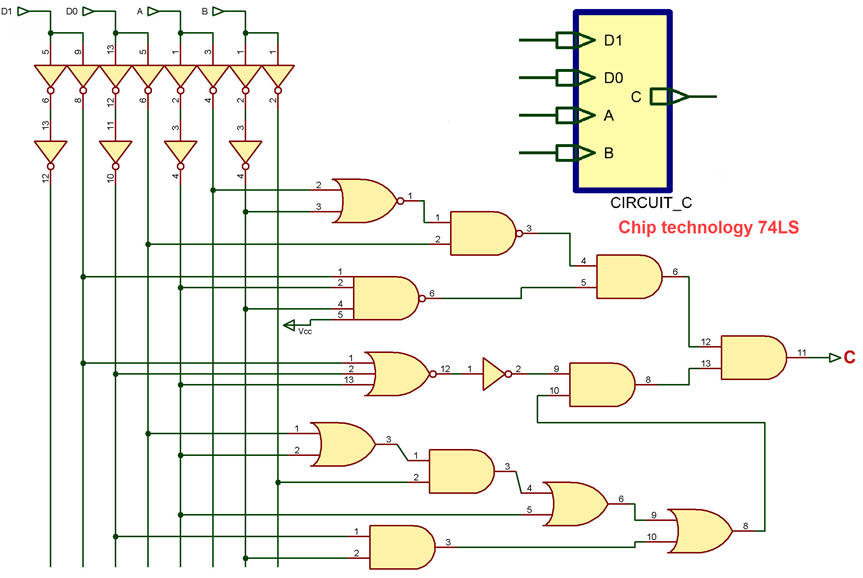Fig. 4. Circuit_C captured in Proteus ready for running simulations.

Picture showing results for given combinations.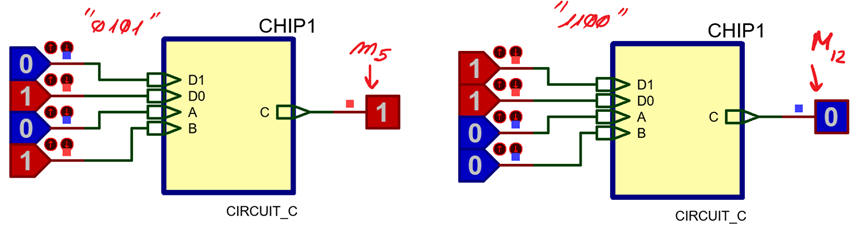Fig. 5. Simulation results when applying input binary combinations "0101" and "1100". We can observe that "0101" generates minterm m5 and "1100" generates maxterm M12.

In this way, trying all 16 combinations we reach the final truth table:2. Method 2 planning

Numerical engine WolframAlpha.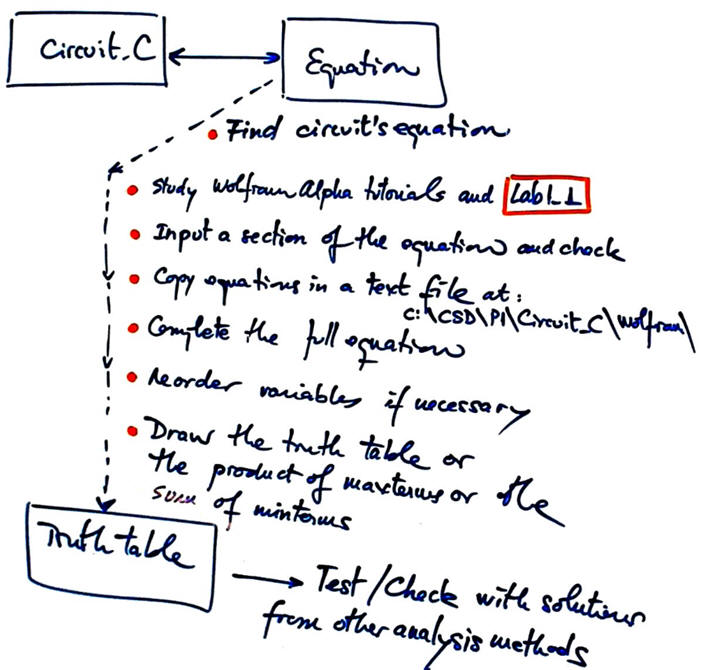Fig. 6. Planning, a bullet list of operations.

- Write the circuit equation in a text file.

- Use files to copy and adapt them from .

- Reorder input variables if such is the case before annotating maxterms and minterms.

- Draw the truth table.

- Project location:

C:\CSD\P1\Circuit_C\Wolfram\ (files)

3. Method 2 development

Circuit and equation are at the same conceptual level.Fig. 7.  Circuit equation.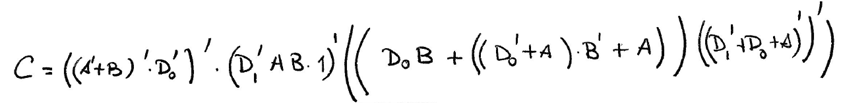This is an example Circuit_C_equation.txt file to be pasted in WolframAlpha window.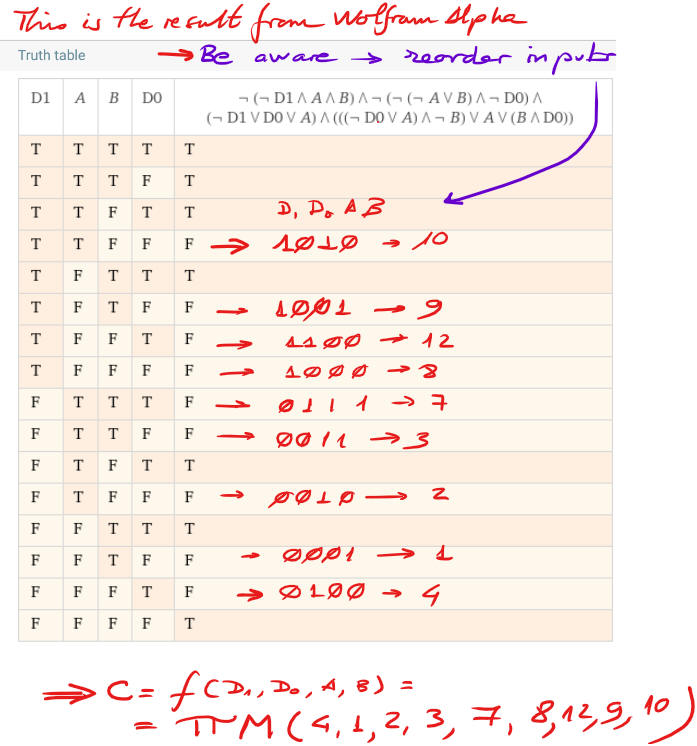Fig. 8. Results representing the truth table.

2. Method 3 planning

Analytical method using Boole's algebra and pen & paper.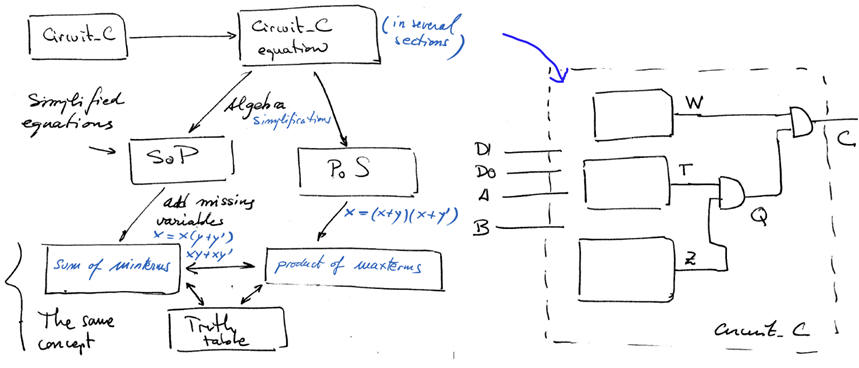Fig. 9. Planning method III subdividing the circuit in sections.

- Find the circuit equation.

- Simplify equations until simple SoP or PoS is obtained.

- Add missing variables to convert SoP into sum of minterms or PoS into product of maxterms.

Project location for saving pictures, scanned sheets of paper, etc...

C:\CSD\P1\Circuit_C\Algebra\(files)

3. Method 3 development

- Handwritten analysis of Circuit_C and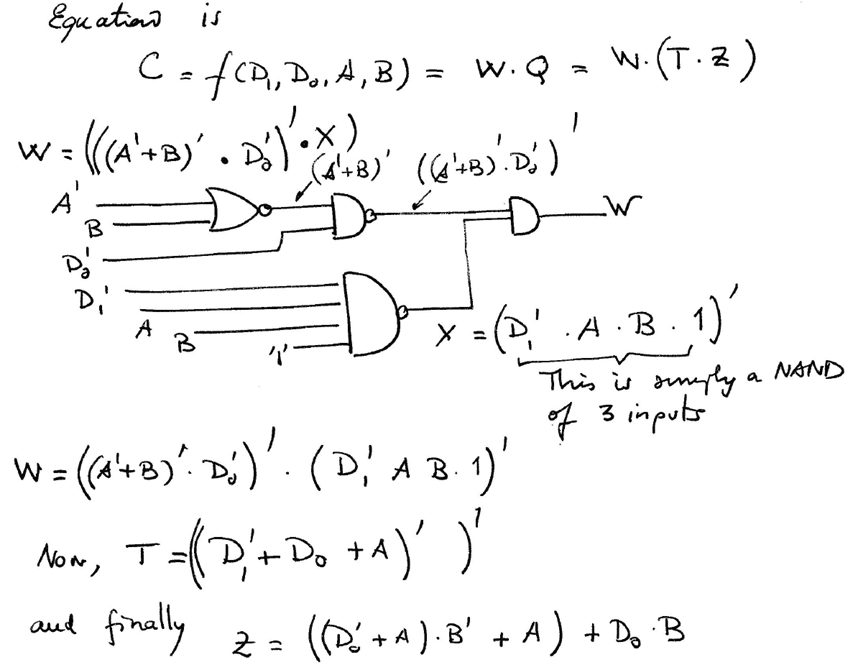Fig. 10. Deducing the circuit's equation.

From circuit equation, we can apply algebra to try to obtain a simple equation.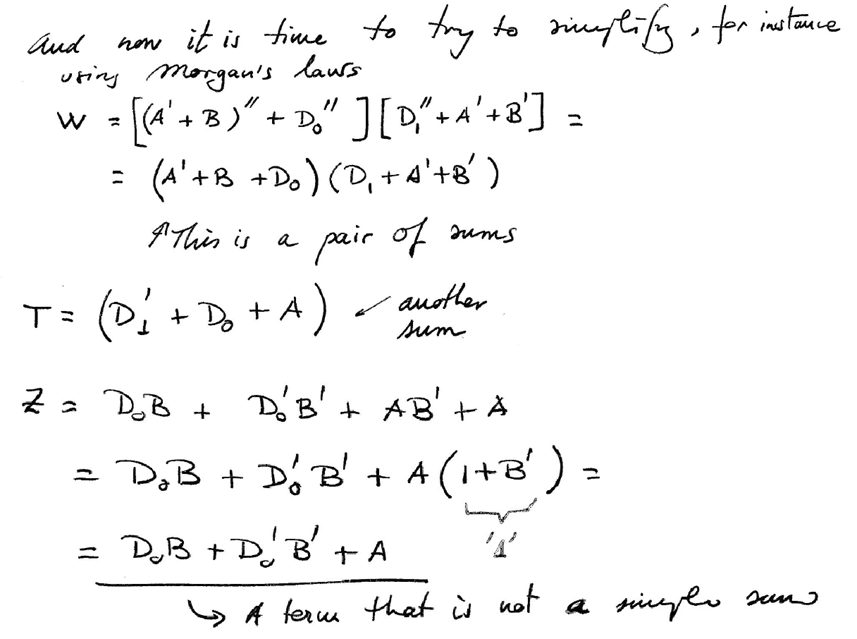Fig. 11. Simplification.

Term Z is complicated, because is not a simple sum or a product. Thus, we can imagine the equation as another independent subcircuit Z = f(D0, A, B):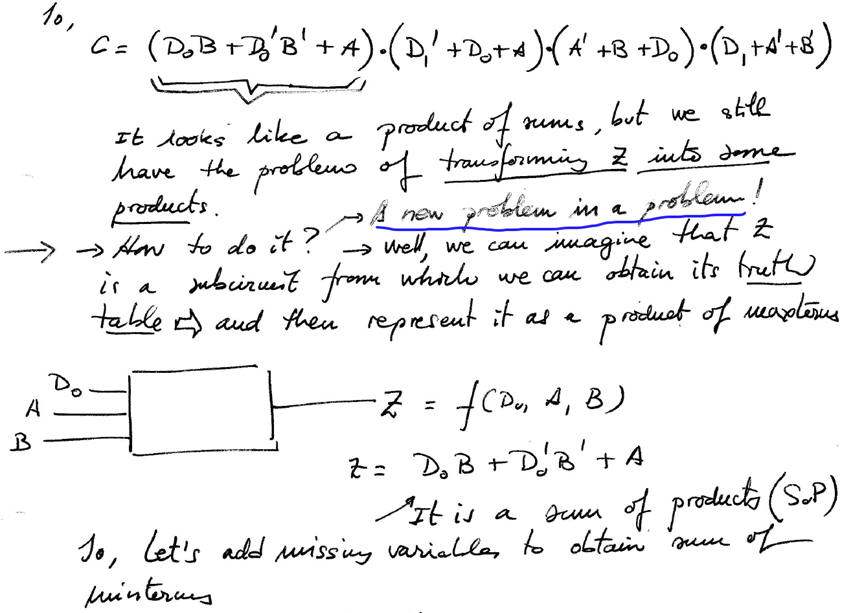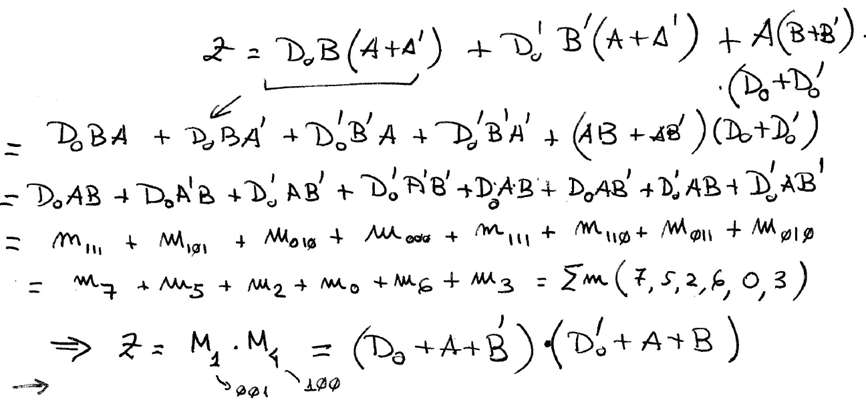Fig. 12. Analysis of Circuit Z = f(D0, A, B).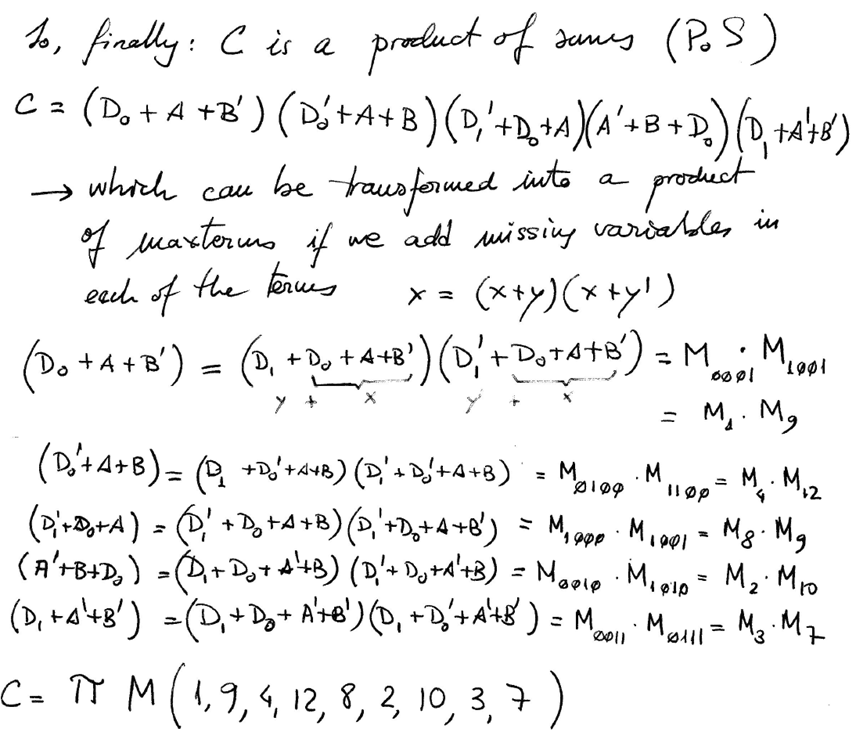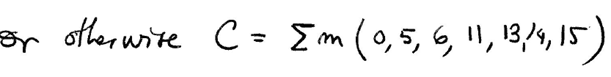Fig. 13. Final equations that we have to compare with results from other methods.

2. Method 4 planning

VHDL synthesis and simulation project.

- Translate to VHDL Circuit_C algebraic equation in Fig. 7 using a similar file.

- Choose a target chip, for instance CPLD MAX II EPM2210F324C3, and start a VHDL synthesis project using an EDA tool, for instance Quartus Prime.

- Examine and print the RTL and technology schematics.

- Start a VHDL simulation project, for instance in ModelSim-Intel FPGA Starter Edition, using a testbench.

Project location:

C:\CSD\P1\Circuit_C\VHDL\(files)

3. Method 4 development

This is an example of Circuit_C.vhd translated to VHDL to synthesise the circuit.

This is an example Circuit_C_tb.vhd VHDL testbench to obtain the circuit's truth table.

4. Testing (Let us verify whether the results are correct)

Testing means to check or verify that the solution is correct and agrees with the initial specifications.

In this analysis section, the simplest way to check the truth table is by comparison with the truth table obtained by other methods.

5. Report

Project report: sheets of paper, scanned and annotated figures, file listings, notes or any other resources. Follow this for writing reports.

6. Prototyping

Any of our circuits can be built as a prototype for laboratory experimentation, measurements and characterisation.